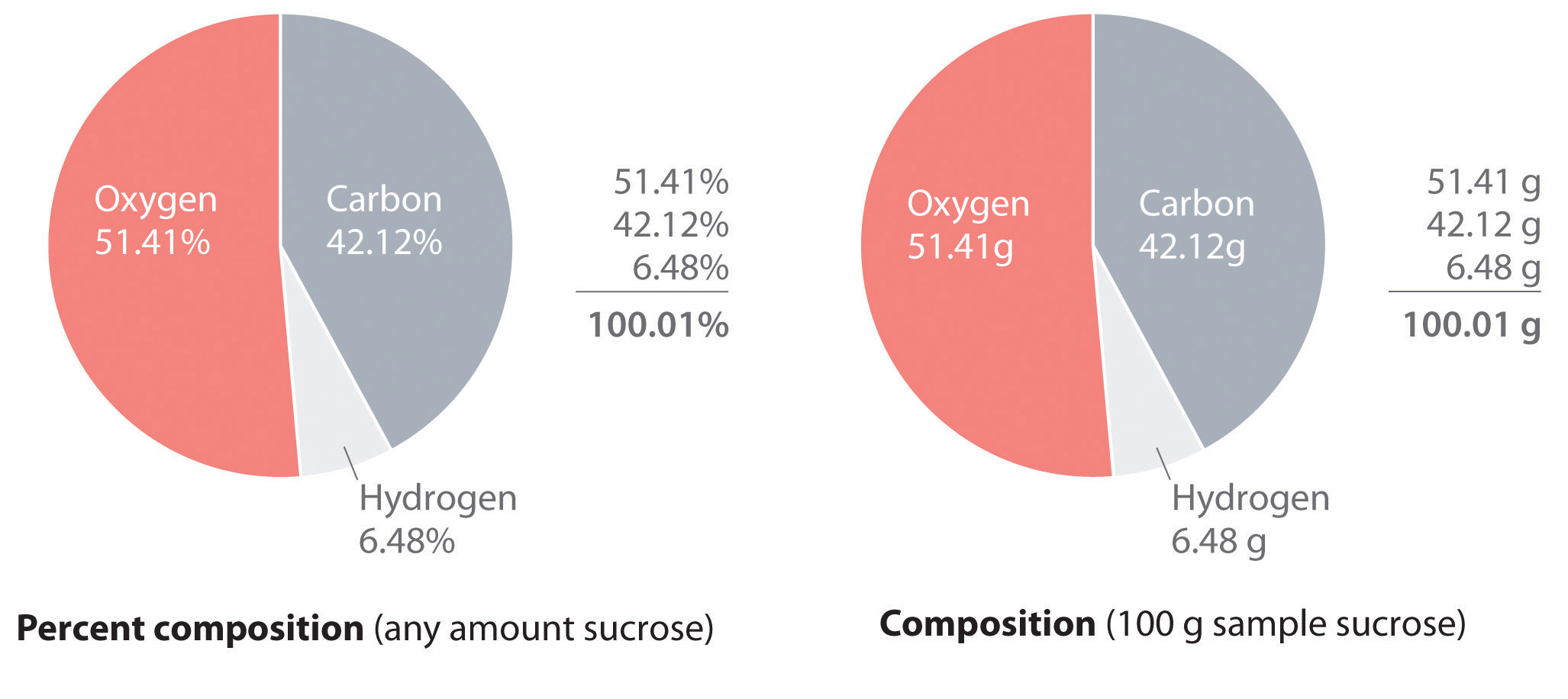# Percentage composition

This is part of preliminary HSC Chemistry course under the topic of Properties of Matter.

### HSC Chemistry Syllabus

• Explore homogeneous mixtures and heterogeneous mixtures through practical investigations:
• Calculating percentage composition by weight of component elements and/or compounds (ACSCH007)

### Percentage Composition

Compounds are substances made up of two or more different elements that are chemically bonded together. The composition of a compound can be described in terms of the percentage by mass of each element in a given sample of the compound.

In industry, obtaining pure metals often requires extracting them from naturally occurring minerals. For example, to extract iron from iron ore, which is typically composed of iron(III) oxide (Fe_2O_3), the percentage composition of iron in the compound must be known.

Suppose we have 100g of iron(III) oxide (Fe_2O_3), and we know that it contains 70g of iron. We can calculate the percentage composition of iron in the compound by dividing the mass of iron by the total mass of the compound and multiplying by 100. In this case, the percentage composition of iron in the iron(III) oxide compound is 70%. Therefore, we can expect to extract 70g of iron from 100g of the compound.

Similarly, let's consider a 88g mass of calcium carbonate (CaCO_3), which is composed of calcium, carbon, and oxygen. If we know that 35 grams of the mass is calcium, 11 grams is carbon, and 42 grams is oxygen, we can calculate the percentage composition of each element in the compound. This calculation involves dividing the mass of each element by the total mass of the compound and multiplying by 100. The percentage composition of calcium, carbon, and oxygen in calcium carbonate is 39.8%, 12.5%, and 47.7%, respectively.

### Calculating Percentage Composition Given Mass

Let's consider the following problem:

Calculate the percentage composition off calcium, carbon, and oxygen in an 88 g sample of calcium carbonate given that 35 grams fit are calcium, 11 grams are carbon, and 42 grams are oxygen

Mass of calcium carbonate = 88 g

Percentage composition of calcium = \frac{35}{88} = 40%

Percentage composition of carbon = \frac{11}{88} = 13%

Percentage composition of oxygen = \frac{42}{88} = 47%

### Calculating Mass from Percentage Composition

Just as we can calculate the percentage composition of a compound given its mass, we can also calculate the mass of each constituent element if we know the percentage composition of the compound.

Let's consider the following problem:

A 152 g sample of the compound Iron (II) Sulfate (Fe_2SO_4). The sample was analysed and it was found to contain 37% iron and 21% sulfur with the remainder of the substance consisting of oxygen. Determine the mass of each element in the compound.

Calculate the mass of each element in the compound using the formula

Mass of compound \times element percentage \divide 100

Mass of Iron: \frac{152 \times 36}{100} = 56 g

Mass of Sulfur: \frac{152 \times 21}{100} = 32 g

Mass of oxygen = Mass of compound – Mass of iron – Mass of sulfur

= 152 – 56 – 32 = 64 g